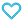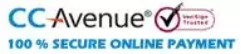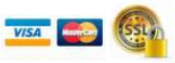# Class-5-Fun Way of Exploring Mathematics

550.00

• Paper Book

The book “Fun way of Exploring Mathematics” is a series of 5 books for Class 1 to 5. The prime motto of creating this series is not only to clear students’ foundational mathematics concepts but also to instill an interest in math from a very young age. To encourage students’ active participation in the classroom, specially curated exercises, projects and activities are incorporated throughout the series. These include the integration of math games into math lessons, connecting math concepts to everyday life, allowing students to solve questions themselves, teamwork encouraging activities, and creating connections among various chapters to reinforce the concepts learned.

873 in stock

Qty:Add to WishlistThe series is in alignment with the NEP 2020 Guidelines which recommend reduced curriculum content to enhance essential learning and critical thinking, experiential learning, curricular integration of essential subjects, skills, and capacities, etc. It also focuses on the multidisciplinary and holistic development of students in both academic and non-academic spheres and puts emphasis on conceptual understanding rather than rote learning. The incorporation of 21st Century skills makes this series helpful in the process of making learning mathematics more holistic for the students.

Key Features –

• Learning Outcomes – Helps to assess the learning objectives of the chapter.
• Let’s Recall – Connects the chapter with lessons learned previously.
• Teacher’s Tips – Contains helpful tips for teachers.
• Fact Box – Consists of general information about the topic learned.
• Let’s Try – Builds confidence in students for self-practice.
• Do you make these mistakes? – Helps prevent mistakes students often do.
• Practice Time – Provides plenty of scope to brush up on the application skills.
• Revision Time – Recapitulate the entire chapter to enhance learning.
• Worksheets – Tests the overall learning outcomes.
• Keywords – Lists the difficult words in each chapter.
• Brain Gym – Enhances critical thinking among students.
• Activity Time – Develops the students’ skills of observation, concentration, teamwork, analyzing, fine motor, creation, etc.
• Word Corner – Enables the students to analyze real-life situations by doing concept and application-based exercises.
• Project – Hands-on project ideas to enhance learning beyond the classroom.
• Subject Integration – Connects mathematics with different subjects.
• SOF IMO Questions – Includes the actual questions from the International Mathematics Olympiad.
Table of Content: 1. Large Numbers 7- digit Numbers, 8-digit and 9-digit Numbers Indian System of Numeration, Reading and Writing 7,8 and 9-digit Numbers International System of Numeration Comparison between Indian and International System of Numeration Face Value and Place Value Expanded Form and Standard (Short) Form Comparison of Numbers, Ascending and Descending Order Predecessor and Successor, Formation of Numbers Rounding Off the Numbers Roman Numerals Revision Time 2. Computation Operations Addition of Large Numbers, Properties of Addition Subtraction of Large Numbers Properties of Subtraction Word Corner 24 Multiplication of Large Numbers Multiplication by 10s, 100s and 1000s Properties of Multiplication Division of Large Numbers Division by 10, 100 and 1000 Properties of Division Word Corner Estimation Revision Time 3. Factors And Multiples Factors, Prime and Composite Numbers Twin Primes, Co-Prime Numbers, Test of Divisibility Prime Factorisation Highest Common Factor (HCF), Methods of Finding HCF Multiples, Least Common Multiple (LCM), Methods of Finding LCM Relationship Between LCM and HCF Word Corner Revision Time WORKSHEET-1 4. Fractions Fractions Types of Fractions Equivalent Fractions, Finding Equivalent Fractions Checking for Equivalent Fractions Reducing a Fraction to Its Lowest Form Comparing and Ordering of Fractions Addition of Fractions Subtraction of Fractions Multiplication of Fractions Division of Fractions Word Corner Revision Time 5. Decimals Decimal Numbers, Place Value Chart Expanding Decimal Numbers Conversion of Decimals Like and Unlike Decimals Comparison of Decimals Addition of Decimals, Subtraction of Decimals Multiplication of Decimals Division of Decimals Word Corner Revision Time 6. Symmetry, Patterns And Nets Symmetry, Reflection Tessellation Slides, Flips and Turns, Rotational Symmetry Patterns Number Patterns Nets Drawing Cube and Cuboid Views of Solid Shapes Revision Time WORKSHEET-2 7. Geometry Point and Line Angle Types of Lines Polygon Triangle, Classification of Triangles Revision Time 8. Perimeter, Area And Volume Perimeter Area Area of a Triangle Area of Irregular Shapes Volume Calculating Volumes Using Formulas Word Corner Revision Time 9. Measurement Metric Measures in Decimal Form, Conversion of Metric Measures Addition and Subtraction of Metric Measures, Multiplication and Division of Metric Measures Word Corner Estimation of Measurement Revision Time WORKSHEET-3 10. Time And Temperature Conversion of Time, Conversion of Higher Units into Lower Units Conversion of Lower Units into Higher Units Calendar Addition and Subtraction of Time Time Duration Finding Number of Days Temperature, Conversion of Temperature Revision Time 11. Mathematics In Daily Life Unitary Method Profit and Loss, Calculating Profit and Loss Calculating Cost Price Calculating Selling Price Map Revision Time 12. Data Handling Circle Graph or Pie Chart Line Graph Tally Marks Revision Time 13. Percentage Ready Percentage Converting a Fraction into Percentage, Converting Percentage into Fraction Converting a Decimal into Percentage, Converting Percentage into Decimal Finding Percentage of a Number or Quantity, What Percent is One Number of Another? Revision Time WORKSHEET-4 Answer Key
ISBN11 9789355556189 MTG Editorial Board 2023-24 236 Class 5 School Books Mathematics 500gm